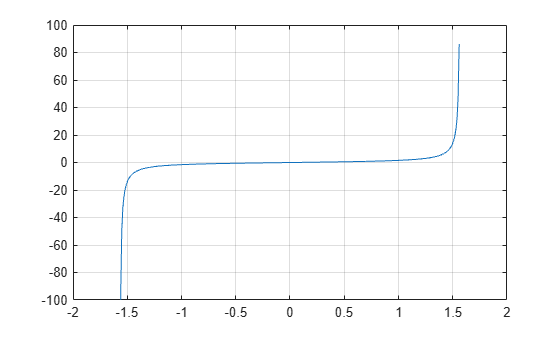tan

Description

example

Y = tan(X) returns the tangent of each element of X. The tan function operates element-wise on arrays. The function accepts both real and complex inputs.

• For real values of X, tan(X) returns real values in the interval [-∞, ∞].

• For complex values of X, tan(X) returns complex values.

Examples

collapse all

Plot the tangent function over the domain $-\pi /2\le x\le \pi /2$ .

x = (-pi/2)+0.01:0.01:(pi/2)-0.01;
plot(x,tan(x)), grid onCalculate the tangent of the complex angles in vector x.

x = [-i pi+i*pi/2 -1+i*4];
y = tan(x)
y = 1×3 complex

0.0000 - 0.7616i  -0.0000 + 0.9172i  -0.0006 + 1.0003i

Input Arguments

collapse all

Input angle in radians, specified as a scalar, vector, matrix, or multidimensional array.

Data Types: single | double
Complex Number Support: Yes

Output Arguments

collapse all

Tangent of input angle, returned as a real-valued or complex-valued scalar, vector, matrix or multidimensional array.

collapse all

Tangent Function

The tangent of an angle, α, defined with reference to a right angled triangle is

.The tangent of a complex argument, α, is

$\text{tan}\left(\alpha \right)=\frac{{e}^{i\alpha }-{e}^{-i\alpha }}{i\left({e}^{i\alpha }+{e}^{-i\alpha }\right)}\text{\hspace{0.17em}}.$

.

Tips

• In floating-point arithmetic, tan is a bounded function. That is, tan does not return values of Inf or -Inf at points of divergence that are multiples of pi, but a large magnitude number instead. This stems from the inaccuracy of the floating-point representation of π.